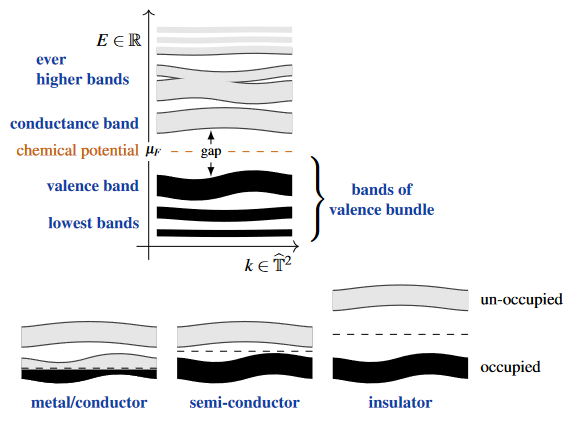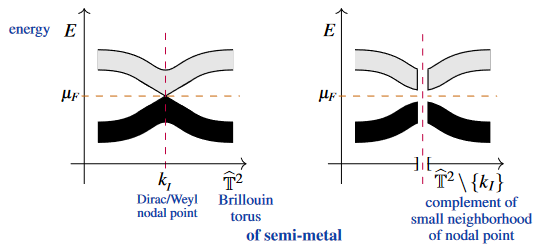Contents

# Contents

## Idea

By Bloch-Floquet theory the available energies of electron-excitations in crystals depends smoothly on the momentum/wave number $k$ of the excitation, taking values in the Brillouin torus, and otherwise only on a discrete index set labelling the remaining “quantum numbers?” (the electron‘s orbitals?, spin. etc.). Fixing the latter values (and jointly denoting them $\mathbf{n}$, say), the given function $k \mapsto E_{\mathbf{n}}(k)$ (or rather its graph) is called the $\mathbf{n}$th electronic band (or just $\mathbf{n}$th band, for short) of the material.

Often, the values of $E_{\mathbf{n}}(-)$ are closely spaced as $\mathbf{n}$ varies in certain subsets of its allowed values. For instance if $\mathbf{n}$ includes the spin of the electrons and if there is a (typically small) spin-orbit coupling and no sizeable external magnetic field, then the energies $E_{n,\uparrow}(-)$ and $E_{n, \downarrow}$ differ (only) slightly. In these cases the graphs of these values jointly look approximately like a single but thickened curve (as such shown in the following schematic graphics), which is where the name “band” originates from.

The band geometry around the electron chemical potential of a material controls its electrical conductivity:(graphics from SS 22)
metal/conductorthe electron chemical potential is inside the valence band
insulatorthe electron chemical potential is inside a large gap between (what is then) the valence- and conduction-band
semi-conductorthe electron chemical potential is inside a small gap between valence and conduction band
semi-metalthere is a large gap between valence and conduction band, except over a codimension$\geq 2$ locus, where the gap closes right at the chemical potential(graphics from SS 22)

### General

Textbook accounts:

• Karlheinz Seeger, Section 2 of: Semiconductor Physics, Advanced texts in physics, Springer (2004) $[$doi:10.1007/978-3-662-09855-4$]$

• Sheng San Li, Energy Band Theory, in S. S. Li, (ed.): Semiconductor Physical Electronics, Springer (2006) 61-104 $[$doi:10.1007/0-387-37766-2_4$]$

Lecture notes:

• David Tong, Chapter 2 of: Lectures on solid state physics (2017) $[$pdf, webpage$]$

Account with focus on topological phases of matter (topological insulators, semimetals etc.):

• Yuejin Guo, Jean-Marc Langlois and William A. Goddard , Electronic Structure and Valence-Bond Band Structure of Cuprate Superconducting Materials, New Series, 239 4842 (1988) 896-899 $[$jstor:1700316$]$
• Jingsan Hu, Jianfei Gu, Weiyi Zhang, Bloch’s band structures of a pair of interacting electrons in simple one- and two-dimensional lattices, Physics Letters A 414 (2021) 127634 $[$doi:10.1016/j.physleta.2021.127634$]$